# Polynomials

## Objective

Classify polynomials through identification of degree and leading coefficient. Graph a polynomial function from a table of values; prove degree using successive differences.

## Common Core Standards

### Core Standards

?

• A.APR.A.1 — Understand that polynomials form a system analogous to the integers, namely, they are closed under the operations of addition, subtraction, and multiplication; add, subtract, and multiply polynomials.

• F.IF.C.7.C — Graph polynomial functions, identifying zeros when suitable factorizations are available, and showing end behavior.

• F.IF.C.9 — Compare properties of two functions each represented in a different way (algebraically, graphically, numerically in tables, or by verbal descriptions). For example, given a graph of one quadratic function and an algebraic expression for another, say which has the larger maximum.

?

• F.BF.B.3

• F.IF.B.4

• F.IF.C.7.A

## Criteria for Success

?

1. Describe that polynomials are classified by the highest degree (exponent) of the function.
2. Describe that the degree of the polynomial indicates shape of the function, and the leading coefficient further indicates the direction of the graph of the function at each extreme.
3. Describe how to identify the degree and leading coefficient of a polynomial from a table of values using successive differences.
4. Explain that parent functions are the basic version of a polynomial function and that the function, like quadratics, can be transformed with translations, reflections, and dilations.
5. Graph multiple functions in a graphing calculator in [Y=] and [GRAPH]. "Turn on" and "turn off" functions in [Y=].
6. Use [STAT] to enter data into L1 and graph functions.

## Anchor Problems

?

### Problem 1

Graph each of the functions in your graphing calculator:

${h(x)=x^2}$

${j(x)=x^3}$

${k(x)=x^4}$

${m(x)=x^5}$

You’ll notice your calculator will look like this: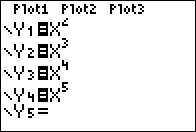### Problem 2

What do these functions have in common? How are they different?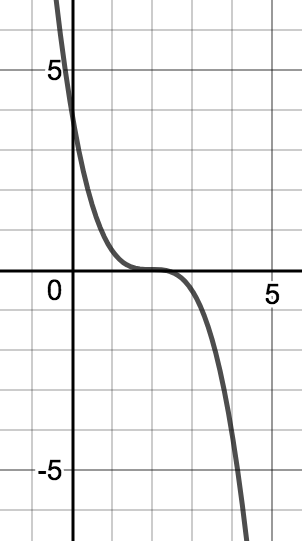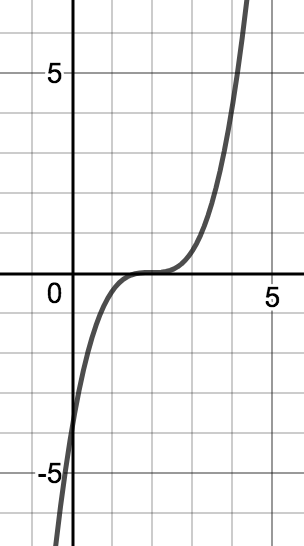${f(x)=-\frac{1}{2}(x-2)^3}$ ${g(x)=\frac{1}{2}(x-2)^3}$

### Problem 3

What is the degree of each of the functions shown in tables? Use a graph of the coordinate points to approximate the shape.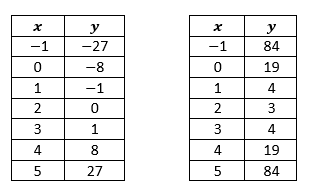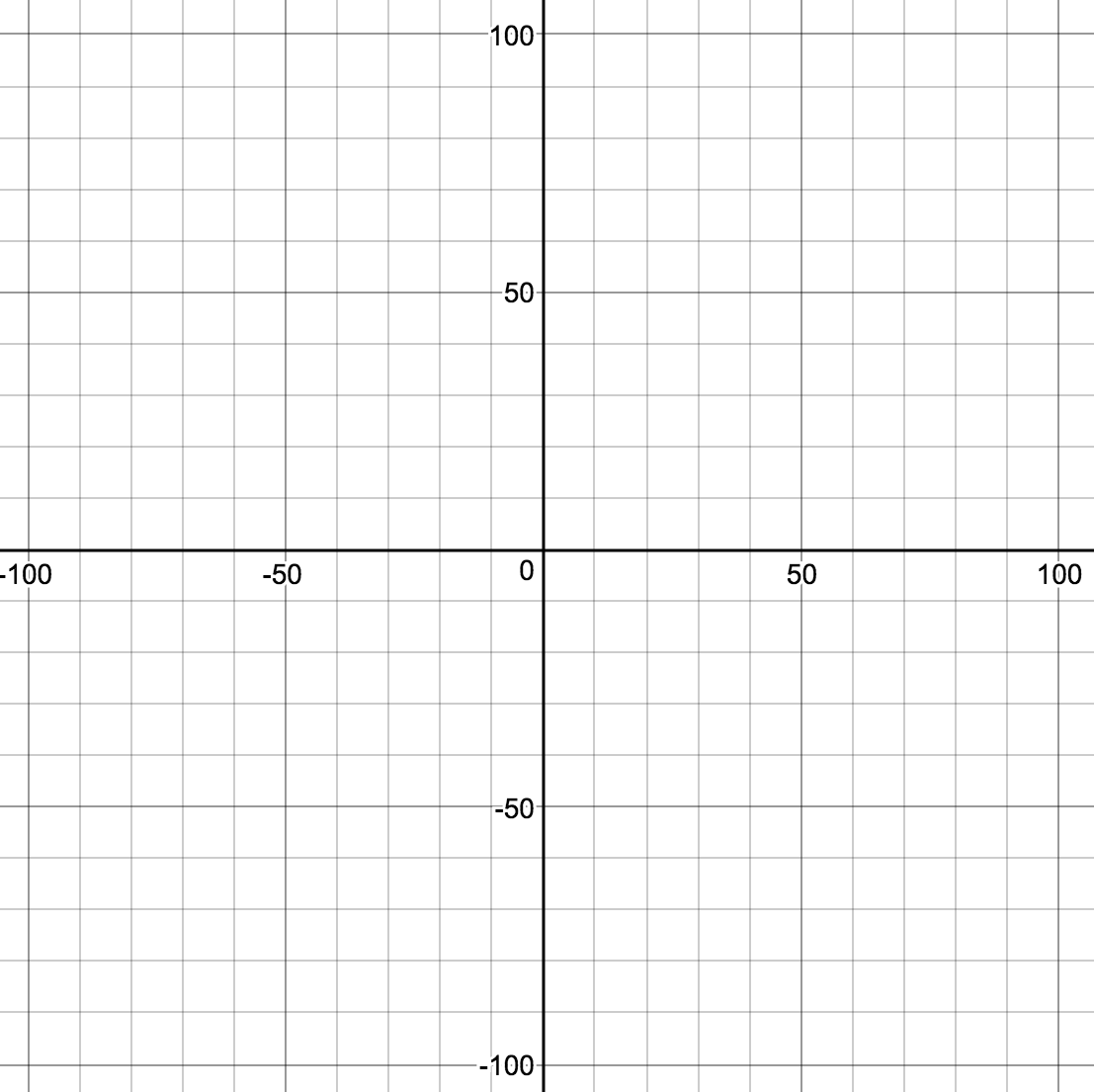## Problem Set

?

The following resources include problems and activities aligned to the objective of the lesson that can be used to create your own problem set.

• Include problems using Anchor Problems #1 and #2 to make a table of values and show the successive differences to illustrate the degree.
• Include problems where students are given an equation and they are asked to graph the function just by choosing points to plug in and approximating the shape. Look for students who are choosing a few points and then filling in others to get more information about the shape.
• Include problems where students are given graphs and asked to identify a possible degree and describe the function. This Desmos link has a good start for ideas.

 $x$ $y$ -7 -245 -4 -74 -1 -11 2 -2 5 7 8 70 11 241